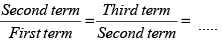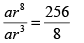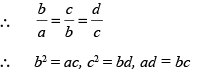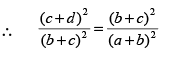Courses

# Geometric Progression CA Foundation Notes | EduRev

## CA Foundation : Geometric Progression CA Foundation Notes | EduRev

The document Geometric Progression CA Foundation Notes | EduRev is a part of the CA Foundation Course Quantitative Aptitude for CA CPT.
All you need of CA Foundation at this link: CA Foundation

Geometric Progression

Let us consider the following sequence of numbers :

(1) 1, 2, 4, 8, 16, .....

(2) 3, 1, 1/3, 1/9, 1/27,.....

(3) 1, – 3, 9, – 27, .....

(4) x, x2 , x, x4 , .......

If we see the patterns of the terms of every sequence in the above examples each term is related to the leading term by a definite rule.

For Example (1), the first term is 1, the second term is twice the first term, the third term is 22 times of the leading term.

Again for Example (2), the first term is 3, the second term is 1/3 times of the first term, third term is 1/32 times of the first term.

A sequence with this property is called a gemetric progression.

A sequence of numbers in which the ratio of any term to the term which immediately precedes is the same non zero number (other than1), is called a geometric progression or simply G. P.

This ratio is called the common ratio.

Thus,is called the common ratio of the geometric progression.

Examples (1) to (4) are geometric progressions with the first term 1, 3, 1,x and with common ratio 2, 1/3, -3 and x respectively.

The most general form of a G. P. with the first term a and common ratio r is a, ar, ar2, ar3,.....

General Term-

Let us consider a geometric progression with the first term a and common ratio r. Then its terms are given by
a, ar, ar2, ar3,.....

In this case,
t1 = a = ar1-1
t2 = ar = ar2-1
t3= ar2 = ar3-1
t4 = ar3 = ar4-1
..... .....

On generalisation, we get the expression for the nth term as

tn = arn-1 ........(A)

Some Properties of a G.P.

(i) If all the terms of a G. P. are multiplied by the same non-zero quantity, the resulting series is also in G. P. The resulting G. P. has the same common ratio as the original one.

If a, b, c, d, ... are in G. P.

then ak, bk, ck, dk ... are also in G. P. ( k ≠ 0)

(ii) If all the terms of a G. P. are raised to the same power, the resulting series is also in G. P.

Let a, b, c, d ... are in G. P.

then ak, bk, ck, dk, ... are also in G. P. ( k ≠ 0 )

The common ratio of the resulting G. P. will be obtained by raising the same power to the original common ratio.

Example 1.  Find the 6th term of the G.P.:  4, 8, 16, ..

Solution : In this case the first term (a) = 4

Common ratio (r) = 8 ÷ 4 = 2

Now using the formula tn = arn–1, we get

t6 = 4 × 2 6–1 = 4 × 32 = 128

Hence, the 6th term of the G. P. is 128.

Example 2. The 4th and the 9th term of a G. P. are 8 and 256 respectively. Find the G.P.

Solution : Let a be the first term and r be the common ratio of the G. P., then

t4 = ar4–1 = ar3

t9 = ar9–1 = ar8

According to the question, ar8 = 256 ....... (1)

and ar3 = 8 ......(2)

∴or r5 = 32 = 25

∴  r = 2

Again from (2), a × 2 3 = 8

∴ a = 8/8 = 1

Therefore,  the G.P. is 1, 2, 4, 8, 16, ...

Example 3. Which term of the G. P.:  5, –10, 20, – 40, ... is 320?

Solution :  In this case, a = 5; r = -10/2 = -5

Suppose that 320 is the nth term of the G. P.

By the formula, tn = arn–1, we get

tn = 5. (–2)n–1

∴ 5. (–2)n–1 = 320     (Given)

∴ (–2)n–1 = 64 = (–2)

∴ n – 1 = 6

∴ n = 7 Hence, 320 is the 7th term of the G. P.

Example 4. If a, b, c, and d are in G. P., then show that (a + b)2, (b + c)2, and (c + d)are also in G. P.

Solution : Since a, b, c, and d are in G. P.,.......(1)

Now, (a + b)2 (c + d)2 = [( a + b ) ( c + d )]2 = (ac + bc + ad + bd)2

= (b2 + c2 + 2bc)2 ...[Using (1)]

= [(b + c)2]2Thus, (a + b)2, (b + c)2, (c + d)2 are in G. P.

Offer running on EduRev: Apply code STAYHOME200 to get INR 200 off on our premium plan EduRev Infinity!

66 docs|68 tests

,

,

,

,

,

,

,

,

,

,

,

,

,

,

,

,

,

,

,

,

,

;